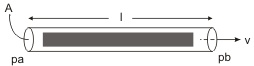﻿ 20-sim webhelp > Library > Iconic Diagrams > Hydraulics > Components > HydraulicInertia

# HydraulicInertia

## Library

Iconic Diagrams\Hydraulics\Components

## Use

Domains: Continuous. Size: 1-D. Kind: Iconic Diagrams (Hydraulics).

## Description

When a fluid flow changes speed, a force is needed to accelerate the fluid. This force is equal to the acceleration multiplied by the mass of the fluid.

F = m * dv/dtThe fluid mass is equal to the pipe area multiplied by the pipe length and the fluid density:

m = A * l * rho

The force will result in a pressure differential:

F = A * dp = A * (pa.p - pb.p)

We can rewrite the formulas as

dp = rho * l * dv/dt

and by introducing the fluid flow phi:

v = phi/A

we get the formula for an hydraulic inertia:

dp = (rho * l / A) * d phi/dt

## Interface

 Ports Description pa, pb Both terminals of the hydraulic inertia. Causality fixed pressure out pa fixed pressure out pb Parameters rho d l Mass density of the fluid [kg/m3]. pipe diameter [m] pipe length [m]